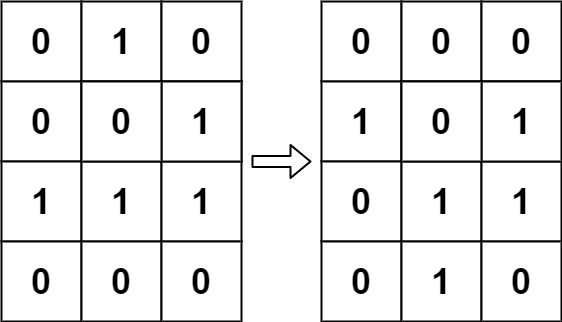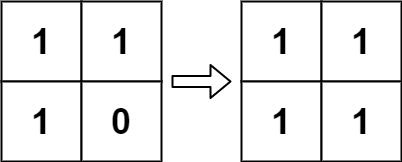# Question

Formatted question description: https://leetcode.ca/all/289.html

According to Wikipedia's article: "The Game of Life, also known simply as Life, is a cellular automaton devised by the British mathematician John Horton Conway in 1970."

The board is made up of an m x n grid of cells, where each cell has an initial state: live (represented by a 1) or dead (represented by a 0). Each cell interacts with its eight neighbors (horizontal, vertical, diagonal) using the following four rules (taken from the above Wikipedia article):

1. Any live cell with fewer than two live neighbors dies as if caused by under-population.
2. Any live cell with two or three live neighbors lives on to the next generation.
3. Any live cell with more than three live neighbors dies, as if by over-population.
4. Any dead cell with exactly three live neighbors becomes a live cell, as if by reproduction.

The next state is created by applying the above rules simultaneously to every cell in the current state, where births and deaths occur simultaneously. Given the current state of the m x n grid board, return the next state.

Example 1:Input: board = [[0,1,0],[0,0,1],[1,1,1],[0,0,0]]
Output: [[0,0,0],[1,0,1],[0,1,1],[0,1,0]]


Example 2:Input: board = [[1,1],[1,0]]
Output: [[1,1],[1,1]]


Constraints:

• m == board.length
• n == board[i].length
• 1 <= m, n <= 25
• board[i][j] is 0 or 1.

• Could you solve it in-place? Remember that the board needs to be updated simultaneously: You cannot update some cells first and then use their updated values to update other cells.
• In this question, we represent the board using a 2D array. In principle, the board is infinite, which would cause problems when the active area encroaches upon the border of the array (i.e., live cells reach the border). How would you address these problems?

# Algorithm

State 0: Dead cells turned into dead cells

State 1: Live cells are transformed into live cells

State 2: Live cells turned into dead cells

State 3: Dead cells transformed into live cells


Take the remainder of 2 for all states

• Then states 0 and 2 become dead cells,
• State 1 and 3 are living cells

Mission complete.

First scan the original array one by one, for each position, scan eight locations around it

• If you encounter state 1 or 2, the counter will be incremented by 1, and 8 neighbors will be scanned
• If there are less than two living cells or more than three living cells, and the current position is a living cell, mark status 2
• If there are exactly three living cells and currently dead cells, mark status 3 After completing the scan, scan the data again, and the remainder of 2 becomes the result we want

# Code

• 
public class Game_of_Life {

class Solution {
public void gameOfLife(int[][] board) {

// Neighbors array to find 8 neighboring cells for a given cell
int[] neighbors = {0, 1, -1};

int rows = board.length;
int cols = board.length;

// Iterate through board cell by cell.
for (int row = 0; row < rows; row++) {
for (int col = 0; col < cols; col++) {

// For each cell count the number of live neighbors.
int liveNeighbors = 0;

for (int i = 0; i < 3; i++) {
for (int j = 0; j < 3; j++) {

if (!(neighbors[i] == 0 && neighbors[j] == 0)) {
int r = (row + neighbors[i]);
int c = (col + neighbors[j]);

// Check the validity of the neighboring cell.
// and whether it was originally a live cell.
if ((r < rows && r >= 0) && (c < cols && c >= 0) && (Math.abs(board[r][c]) == 1)) {
liveNeighbors += 1;
}
}
}
}

// Rule 1 to Rule 3
if ((board[row][col] == 1) && (liveNeighbors < 2 || liveNeighbors > 3)) {
// -1 signifies the cell is now dead but originally was live.
board[row][col] = -1;
}
// Rule 4
if (board[row][col] == 0 && liveNeighbors == 3) {
// 2 signifies the cell is now live but was originally dead.
board[row][col] = 2;
}
}
}

// Get the final representation for the newly updated board.
for (int row = 0; row < rows; row++) {
for (int col = 0; col < cols; col++) {
if (board[row][col] > 0) {
board[row][col] = 1;
} else {
board[row][col] = 0;
}
}
}
}
}
}

############

class Solution {
public void gameOfLife(int[][] board) {
int m = board.length, n = board.length;
int[][] dirs
= new int[][] { {0, -1}, {0, 1}, {1, 0}, {-1, 0}, {1, 1}, {1, -1}, {-1, 1}, {-1, -1}};
for (int i = 0; i < m; ++i) {
for (int j = 0; j < n; ++j) {
int cnt = 0;
for (int[] dir : dirs) {
int x = i + dir, y = j + dir;
if (x >= 0 && x < m && y >= 0 && y < n
&& (board[x][y] == 1 || board[x][y] == 2)) {
++cnt;
}
}
if (board[i][j] == 1 && (cnt < 2 || cnt > 3)) {
board[i][j] = 2;
} else if (board[i][j] == 0 && cnt == 3) {
board[i][j] = 3;
}
}
}
for (int i = 0; i < m; ++i) {
for (int j = 0; j < n; ++j) {
board[i][j] %= 2;
}
}
}
}

• // OJ: https://leetcode.com/problems/game-of-life/
// Time: O(N^2)
// Space: O(1)
class Solution {
public:
void gameOfLife(vector<vector<int>>& A) {
int M = A.size(), N = A.size();
auto alive = [&](int x, int y) {
return x >= 0 && x < M && y >= 0 && y < N && (A[x][y] & 1);
};
for (int i = 0; i < M; ++i) {
for (int j = 0; j < N; ++j) {
int cnt = 0;
for (int dx = -1; dx <= 1; ++dx) {
for (int dy = -1; dy <= 1; ++dy) {
if (dx == 0 && dy == 0) continue;
cnt += alive(i + dx, j + dy);
}
}
if (cnt == 3 || (A[i][j] && cnt == 2)) A[i][j] |= 2;
}
}
for (int i = 0; i < M; ++i) {
for (int j = 0; j < N; ++j) A[i][j] >>= 1;
}
}
};

• class Solution:
def gameOfLife(self, board: List[List[int]]) -> None:
"""
Do not return anything, modify board in-place instead.
"""
m, n = len(board), len(board)
dirs = [[-1, 0], [1, 0], [0, -1], [0, 1], [-1, -1], [-1, 1], [1, -1], [1, 1]]
for i in range(m):
for j in range(n):
cnt = sum(
1
for a, b in dirs
if 0 <= i + a < m
and 0 <= j + b < n
and board[i + a][j + b] in (1, 2)
)
if board[i][j] == 1 and (cnt < 2 or cnt > 3):
board[i][j] = 2
elif board[i][j] == 0 and (cnt == 3):
board[i][j] = 3
for i in range(m):
for j in range(n):
board[i][j] %= 2

############

class Solution(object):
def gameOfLife(self, board):
"""
:type board: List[List[int]]
:rtype: void Do not return anything, modify board in-place instead.
"""

def helper(board, p, q):
cnt = 0
for i in range(p - 1, p + 2):
for j in range(q - 1, q + 2):
if i == p and j == q:
continue
if 0 <= i < len(board) and 0 <= j < len(board) and board[i][j] & 1:
cnt += 1
if cnt == 3 or (board[p][q] == 1 and cnt == 2):
board[p][q] |= 2

for i in range(0, len(board)):
for j in range(0, len(board)):
helper(board, i, j)

for i in range(0, len(board)):
for j in range(0, len(board)):
board[i][j] >>= 1


• func gameOfLife(board [][]int) {
m, n := len(board), len(board)
dirs := int{ {0, -1}, {0, 1}, {1, 0}, {-1, 0}, {1, 1}, {1, -1}, {-1, 1}, {-1, -1} }
for i := 0; i < m; i++ {
for j := 0; j < n; j++ {
cnt := 0
for _, dir := range dirs {
x, y := i+dir, j+dir
if x >= 0 && x < m && y >= 0 && y < n && (board[x][y] == 1 || board[x][y] == 2) {
cnt++
}
}
if board[i][j] == 1 && (cnt < 2 || cnt > 3) {
board[i][j] = 2
} else if board[i][j] == 0 && cnt == 3 {
board[i][j] = 3
}
}
}
for i := 0; i < m; i++ {
for j := 0; j < n; j++ {
board[i][j] %= 2
}
}
}

• /**
Do not return anything, modify board in-place instead.
*/
function gameOfLife(board: number[][]): void {
const m = board.length;
const n = board.length;
for (let i = 0; i < m; ++i) {
for (let j = 0; j < n; ++j) {
let live = -board[i][j];
for (let x = i - 1; x <= i + 1; ++x) {
for (let y = j - 1; y <= j + 1; ++y) {
if (x >= 0 && x < m && y >= 0 && y < n && board[x][y] > 0) {
++live;
}
}
}
if (board[i][j] === 1 && (live < 2 || live > 3)) {
board[i][j] = 2;
}
if (board[i][j] === 0 && live === 3) {
board[i][j] = -1;
}
}
}
for (let i = 0; i < m; ++i) {
for (let j = 0; j < n; ++j) {
if (board[i][j] === 2) {
board[i][j] = 0;
}
if (board[i][j] === -1) {
board[i][j] = 1;
}
}
}
}


• public class Solution {
public void GameOfLife(int[][] board) {
int m = board.Length;
int n = board.Length;
for (int i = 0; i < m; ++i) {
for (int j = 0; j < n; ++j) {
int live = -board[i][j];
for (int x = i - 1; x <= i + 1; ++x) {
for (int y = j - 1; y <= j + 1; ++y) {
if (x >= 0 && x < m && y >= 0 && y < n && board[x][y] > 0) {
++live;
}
}
}
if (board[i][j] == 1 && (live < 2 || live > 3)) {
board[i][j] = 2;
}
if (board[i][j] == 0 && live == 3) {
board[i][j] = -1;
}
}
}
for (int i = 0; i < m; ++i) {
for (int j = 0; j < n; ++j) {
if (board[i][j] == 2) {
board[i][j] = 0;
}
if (board[i][j] == -1) {
board[i][j] = 1;
}
}
}
}
}